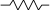# Raymii.orgQuis custodiet ipsos custodes?
Home | About | All pages | Cluster Status | RSS Feed | Gopher

## std::accumulate in C++

Published: 23-10-2019 | Last update: 07-11-2020 | Author: Remy van Elst | Text only version of this article

❗ This post is over three years old. It may no longer be up to date. Opinions may have changed.

I'm using codewars to practice my development skills. Today I found out about the `std::accumulate` method in C++ while doing an exercise there. I'm sharing it here because I never heard of it before. It is the `<numeric>` header, and it also accepts a custom binary function to apply instead of `operator+`. This snippet shows some examples including a lambda operator and the for loop you would use otherwise.

Recently I removed all Google Ads from this site due to their invasive tracking, as well as Google Analytics. Please, if you found this content useful, consider a small donation using any of the options below:

I'm developing an open source monitoring app called Leaf Node Monitoring, for windows, linux & android. Go check it out!

Consider sponsoring me on Github. It means the world to me if you show your appreciation and you'll help pay the server costs.

You can also sponsor me by getting a Digital Ocean VPS. With this referral link you'll get \$100 credit for 60 days.

### Plain old loop

It performs a fold on a given range. Or, in my case, it gives the sum of all int's in a vector without a loop. Complete documentation here.

Consider this code:

``````std::vector <int> numbers  { 20, 10, -8, 10, 10, 15, 35 };
int sum = 0;
for (auto const& number: numbers)
{
sum += number;
}
std::cout << "sum: " << sum;
``````

The output after compiling is:

``````# C:\Users\Remy\CLionProjects\codewars\cmake-build-debug\src\CodeWars.exe

sum: 92
``````

This can be written shorter using the `accumulate` function:

``````int sum = std::accumulate(numbers.begin(), numbers.end(), 0);
``````

`std::accumulate` accepts an initial value, so to add 10 to the result of the above sum without an intermidiate variable:

``````std::vector <int> numbers  { 20, 10, -8, 10, 10, 15, 35 };
int sum = std::accumulate(numbers.begin(), numbers.end(), 10);
``````

The result will be `102`.

### Lambdas

`std::accumulate` also accepts a function to perform as the fold operator. For example, if you need to get a sum of the result and also multiply every number by 8, in the odd case you need to go from bytes to bits:

``````std::vector <int> numbers  { 10, 20, 30 };
int sum = std::accumulate(numbers.begin(),
numbers.end(),
0,
[](int a, int b) { return a + (b*8);}
);
std::cout << "sum: " << sum;
``````

Output:

``````sum: 480
``````

The binary operator takes the current accumulation value a (initialized to init) and the value of the current element b.

This is a simple example of course, but by using a lambda you can do all kinds of crazy stuff.

From the documentation, on reversing the order:

``````std::accumulate performs a left fold. In order to perform a right fold,
one must reverse the order of the arguments to the binary operator, and
use reverse iterators.
``````
Tags: c++ , codewars , cpp , development , snippets